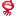# oracle中执行字符串sql语句,Oracle SQL 字符串操作

VARCHAR2数值被限制为2000字符(ORACLE

8中为4000字符)

CHAR数值被限制为255字符(在ORACLE8中是2000)

long类型为2GB

Clob类型为4GB

1、CHR

2、CONCAT

CONCAT(string1,string2)

3、INITCAP

4、LTRIM

5、NLS_INITCAP

'NLS_SORT=sort'

6、NLS_LOWER

Nlsparams参数的形式与用途和NLS_INITCAP中的nlsparams参数是相同的。如果nlsparams没有被包含，那么NLS_LOWER所作的处理和

LOWER相同。

7、NLS_UPPER

8、REPLACE

search_str都将被删除。REPLACE是TRANSLATE所提供的功能的一个子集。

10、RTRIM

RTRIM(string1,[,string2])

string2被缺省设置为单个的空格.数据库将扫描string1,从右边开始.当遇

11、SOUNDEX

12、SUBSTR

SUBSTR(string,a[,b])

13、TRANSLATE

TRANSLATE(string,from_str,to_str)

TRANSLATE是REPLACE所提供的功能的一个超集.

to_str不能为空

.Oracle把空字符串认为是NULL,并且如果TRANSLATE中的任何参数为NULL,那么结果也是NULL.

14、UPPER

16、ASCII

ASCII得到给定字符的字符编码.

17、INSTR

string2[a,b])

string1时从左边开始检查的,开始的位置为a,如果a是一个负数,那么string1是从右边开始进行

a和b都缺省设置为1,这将会返回在string1中第一次出现string2的位置.如果string2在a和b的规定下没有

18、INSTRB

string2[a,[b]])

19、LENGTH

20、LENGTHB

21、NLSSORT

NLSSORT(string[,nlsparams])

Nlsparams的作用和

NLS_INITCAP中的相同.如果忽略参数,会话使用缺省排序.

select

substr(t.cm_node,0,instr(t.cm_node,'/',-1)) from cm_node

t;

CREATE OR REPLACE PACKAGE

PKG_STR_CONV AS

--判断输入的字符串是否全部都是数字

Function isnumber(str varchar2 )

RETURN NUMBER;

--判断输入的字符串是否全部是汉字

Function ischinese(str varchar2 )

RETURN VARCHAR2;

--保留字符串中全部数字

FUNCTION get_number(theStr IN

VARCHAR2 DEFAULT NULL) RETURN VARCHAR2;

--去掉字符串中的开头和结尾的非数字字符

FUNCTION start_end_number(theStr

IN VARCHAR2 DEFAULT NULL) RETURN VARCHAR2;

--将字符串中所有的非汉字字符去掉

FUNCTION trim_chars (theStr IN

VARCHAR2) RETURN VARCHAR2;

--取出字符串中第一个汉字的位置

FUNCTION count_chars (theStr IN

VARCHAR2) RETURN VARCHAR2;

--统计子字符串在主字符串中出现的频率

FUNCTION freq_instr1(string_in IN

VARCHAR2,substring_in IN VARCHAR2,match_case_in IN VARCHAR2

:='IGNORE') RETURN NUMBER;

--从第一个字符串中去除第二个字符串中的字符

Function cutstr(p_FirstStr

VARCHAR2,p_SecondStr VARCHAR2) RETURN VARCHAR2;

--把第一个字符串按第二个字符串中字符的顺序排列的函数

FUNCTION sortstr (p_Str

VARCHAR2,p_SecondStr VARCHAR2) RETURN VARCHAR2;

--拆分字符串,插入测试表的的存储过程

PROCEDURE insert_demo(p_INSERT_STR

VARCHAR2);

--合并字符串的函数

function str_list2(key_name in

varchar2,key in varchar2,coname in varchar2,tname IN varchar2 )

return VARCHAR2;

--删除字符串中的重复字符

function delre(p_str varchar2,

p_token char := ',') return VARCHAR2;

--字段名和值均以字符串形式保存到第三方表的数据集

--函数递归执行实现表达式

END pkg_str_conv;

/

CREATE OR REPLACE PACKAGE BODY

PKG_STR_CONV AS

--判断输入的字符串是否全部都是数字

Function isnumber(str varchar2

)

RETURN NUMBER

IS

n NUMBER;

BEGIN

SELECT to_number(str) INTO n FROM

dual;

return 1;

EXCEPTION

WHEN OTHERS THEN

return 0;

END;

--判断输入的字符串是否全部都是汉字

Function ischinese(str varchar2

)

RETURN VARCHAR2

IS

flag VARCHAR2(5);

BEGIN

SELECT

decode(lengthb(str),2*length(str),'true','false') INTO flag from

dual;

RETURN flag;

EXCEPTION

WHEN OTHERS THEN

RETURN NULL;

END;

--保留字符串中全部数字

FUNCTION get_number(theStr IN

VARCHAR2 DEFAULT NULL) RETURN VARCHAR2

AS

CURR_CHAR VARCHAR2(3);

rtVal VARCHAR2(4000);

BEGIN

rtVal:=NULL;

IF theStr IS NOT NULL

THEN

FOR i IN 1..LENGTH(theStr)

LOOP

CURR_CHAR:=SUBSTR(theStr,i,1);

--ASCII(0..9)=48..57

IF ASCII(CURR_CHAR) BETWEEN 48 AND

57 THEN

rtVal:=rtVal ||

CURR_CHAR;

END IF;

END LOOP;

END IF;

RETURN rtVal;

EXCEPTION

WHEN OTHERS THEN RETURN

NULL;

END;

--去掉字符串中的开头和结尾的非数字字符

FUNCTION start_end_number(theStr

IN VARCHAR2 DEFAULT NULL)

RETURN VARCHAR2 AS

CURR_CHAR VARCHAR2(2);

rtVal VARCHAR2(4000);

BEGIN

rtVal :=

LTRIM(RTRIM(thestr));

IF rtVal IS NOT NULL

THEN

FOR i IN 1 .. LENGTH(rtVal)

LOOP

CURR_CHAR := SUBSTR(rtVal, 1,

1);

IF ISNUMBER(CURR_CHAR) = 0

THEN

rtVal := SUBSTR(rtVal,

2);

ELSE

FOR i IN 1 .. LENGTH(rtVal)

LOOP

CURR_CHAR := SUBSTR(rtVal, -1,

1);

IF ISNUMBER(CURR_CHAR) = 0

THEN

rtVal := SUBSTR(rtVal, 1,

LENGTH(rtVal) - 1);

ELSE

RETURN rtVal;

END IF;

END LOOP;

END IF;

END LOOP;

END IF;

RETURN rtVal;

EXCEPTION

WHEN OTHERS THEN

RETURN NULL;

END START_END_NUMBER;

--将字符串中所有的非汉字字符去掉

FUNCTION trim_chars (theStr IN

VARCHAR2) RETURN VARCHAR2

IS

rtVal VARCHAR2(4000);

CURR_CHAR VARCHAR2(2);

STR VARCHAR2(4000);

BEGIN

rtVal:='';

STR:=theStr;

IF STR IS NOT NULL THEN

FOR i IN 1..LENGTH(STR)

LOOP

CURR_CHAR:=SUBSTR(STR,i,1);

IF LENGTHB(CURR_CHAR)=2

THEN

rtVal:=rtVal ||

CURR_CHAR;

END IF;

END LOOP;

END IF;

RETURN rtVal;

EXCEPTION

WHEN OTHERS THEN RETURN

NULL;

END;

--取出字符串中第一个汉字的位置

FUNCTION count_chars (theStr IN

VARCHAR2) RETURN VARCHAR2

IS

count number(38);

CURR_CHAR VARCHAR2(2);

STR VARCHAR2(4000);

BEGIN

STR:=rtrim(ltrim(theStr));

IF STR IS NOT NULL THEN

FOR i IN 1..LENGTH(STR)

LOOP

CURR_CHAR:=SUBSTR(STR,i,1);

IF LENGTHB(CURR_CHAR)=2

THEN

count:=i;

END IF;

END LOOP;

END IF;

EXCEPTION

WHEN OTHERS THEN RETURN

NULL;

END;

--统计子字符串在主字符串中出现的频率

FUNCTION freq_instr1(string_in IN

VARCHAR2,substring_in IN VARCHAR2,match_case_in IN VARCHAR2 :=

'IGNORE') RETURN NUMBER AS

search_loc NUMBER := 1;

substring_len NUMBER := LENGTH

(substring_in);

check_again BOOLEAN :=

TRUE;

return_value NUMBER :=

0;

BEGIN

IF string_in IS NOT NULL AND

substring_in IS NOT NULL

THEN

WHILE check_again

LOOP

IF UPPER (match_case_in) =

'IGNORE'

THEN

search_loc :=

INSTR (UPPER

(string_in),

UPPER (substring_in), search_loc,

1);

ELSE

search_loc := INSTR (string_in,

substring_in, search_loc, 1);

END IF;

check_again := search_loc

> 0;

IF check_again

THEN

return_value := return_value +

1;

search_loc := search_loc +

substring_len;

END IF;

END LOOP;

END IF;

RETURN return_value;

END freq_instr1;

--函数功能:CutStr(从第一个字符串中去除第二个字符串中的字符的函数)

--传入参数:p_FirstStr-第一个字符串,p_SecondStr-第二个字符串

--功能说明:从第一个字符串中去除第二个字符串中的字符

--详细说明:循环取出第二个字符串中的字符，如果该字符在第一个字符串中存在，则从第一个字符串中去除该字符，并返回剩下部分字符串

FUNCTION cutstr(p_FirstStr IN

VARCHAR2,p_SecondStr IN VARCHAR2) RETURN VARCHAR2

IS

l_StrLength INT;

l_CurrentChar

VARCHAR2(2);

l_ReturnStr

VARCHAR2(4000);

BEGIN

l_ReturnStr:=p_FirstStr;

l_StrLength:=length(p_SecondStr);

WHILE l_StrLength>0

LOOP

l_CurrentChar:=substr(p_SecondStr,l_StrLength,1);

l_ReturnStr:=replace(l_ReturnStr,l_CurrentChar,'');

l_StrLength:=l_StrLength-1;

END LOOP;

RETURN l_ReturnStr;

END;

--函数功能:SortStr(把第一个字符串按第二个字符串中字符的顺序排列的函数)

--传入参数:p_Str-第一个字符串,

p_SecondStr-第二个字符串

--功能说明:把第一个字符串按第二个字符串中字符的顺序排列的函数

--详细说明:顺序取出第二个字符串中的在第一个字符串的字符组合，并返回该字符组合

FUNCTION sortstr ( p_Str

VARCHAR2,p_SecondStr VARCHAR2) RETURN VARCHAR2

IS

l_StrLength INT;

l_ReturnStr

VARCHAR2(4000):='';

l_CurrentChar

VARCHAR2(2);

BEGIN

l_StrLength:=length(p_SecondStr);

WHILE l_StrLength>0

LOOP

l_CurrentChar:=substr(p_SecondStr,l_StrLength,1);

IF

instr(p_Str,l_CurrentChar)>0 THEN

l_ReturnStr:=l_CurrentChar||l_ReturnStr;

END IF;

l_StrLength:=l_StrLength-1;

END LOOP;

RETURN l_ReturnStr;

END;

--字符串的拆分

PROCEDURE insert_demo(p_INSERT_STR

VARCHAR2)

--简单的拆分sql就可以解决

--调用:EXEC

INSERT_DEMO('001￥12￥13，002￥22￥23，003￥32￥33');

--查询:SELECT * FROM

CSDN_DEMO;

AS

v_STR_TEMP

VARCHAR2(502);

v_STR_CURR

VARCHAR2(500);

v_STR_INSERT_1 VARCHAR2(100) :=

'INSERT INTO CSDN_DEMO (ID, FIELD_1, FIELD_2) VALUES (

';

v_STR_INSERT_2

VARCHAR2(500);

BEGIN

IF p_INSERT_STR IS NULL

THEN

DBMS_OUTPUT.PUT_LINE('输入参数为空！');

END IF;

v_STR_TEMP :=

SUBSTR(p_INSERT_STR,1,250) || '，';

LOOP

v_STR_CURR :=

SUBSTR(v_STR_TEMP,1,INSTR(v_STR_TEMP,'，')-1) || '￥';

v_STR_INSERT_2 := NULL;

LOOP

v_STR_INSERT_2 := v_STR_INSERT_2

|| ''''||SUBSTR(v_STR_CURR,1,INSTR(v_STR_CURR,'￥')-1) ||

''',';

v_STR_CURR :=

SUBSTR(v_STR_CURR,INSTR(v_STR_CURR,'￥')+1);

EXIT WHEN v_STR_CURR IS

NULL;

END LOOP;

v_STR_INSERT_2 :=

SUBSTR(v_STR_INSERT_2,1,LENGTH(v_STR_INSERT_2)-1);

v_STR_INSERT_2 := v_STR_INSERT_2

|| ')';

EXECUTE IMMEDIATE v_STR_INSERT_1

|| v_STR_INSERT_2;

v_STR_TEMP :=

SUBSTR(v_STR_TEMP,INSTR(v_STR_TEMP,'，')+1);

EXIT WHEN v_STR_TEMP IS

NULL;

END LOOP;

EXCEPTION

WHEN OTHERS THEN

DBMS_OUTPUT.PUT_LINE(SUBSTR('ERROR:'||SQLERRM,1,255));

END ;

--字符串的合并

--进行测试:select

test.country

function str_list2( key_name in

varchar2,

key in varchar2,

coname in varchar2,

tname in varchar2 )

return varchar2

as

type rc is ref cursor;

str varchar2(4000);

sep varchar2(2);

val varchar2(4000);

cur rc;

begin

open cur for 'select

'||coname||'

from '|| tname || '

where ' || key_name || ' = :x

'

using key;

loop

fetch cur into val;

exit when cur%notfound;

str := str || sep ||

val;

sep := ', ';

end loop;

close cur;

return str;

end;

--删除字符串中的重复字符:

--测试:select

hashset('AA,AA,CC,BB,CC,AA,DD,CC,FF,CC',',')

a,hashset('1,1,1,2,2,2,3,3,4,5,6,7,8,9,123,234,......') b from

dual;

function delre(p_str varchar2,

p_token char := ',')

return varchar2 as

type t_tab is table of int index

by varchar2(60);

l_tab t_tab;

l_n pls_integer := 0;

l_vc varchar2(500) :=

p_str;

idx varchar2(60);

begin

if substr(l_vc, -1, 1)

<> p_token then

l_vc := l_vc ||

p_token;

end if;

loop

l_n := instr(l_vc,

p_token);

exit when(nvl(l_n, 0) =

0);

l_tab(ltrim(rtrim(substr(l_vc, 1,

l_n - 1)))) := 0;

l_vc := substr(l_vc, l_n +

1);

end loop;

idx := l_tab.first;

while idx is not null

loop

l_vc := l_vc || p_token ||

idx;

idx := l_tab.next(idx);

end loop;

return substr(l_vc, 2);

end;

END pkg_str_conv;12-12
07-04788

04-165619
08-06309
01-153253
07-101万+
04-02171
09-26478
04-0399
06-03697
04-07107
09-292620
11-07490
04-04926
02-13588
04-02666
09-02357
06-04536
04-0357

### “相关推荐”对你有帮助么？

•非常没帮助
•没帮助
•一般
•有帮助
•非常有帮助点击重新获取扫码支付余额充值